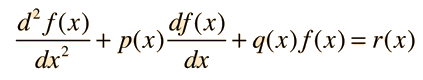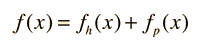Second Order Non-homogeneous Differential Equation

Many physical problems involve second order differential equations. Some applications involve homogeneous equations, but the more general case is the non-homogeneous equation. The general form of the second order differential equation isThe path to a general solution involves finding a solution fh(x) to the homogeneous equation, and then finding a particular solution fp(x) to the non-homogeneous equation (i.e., find any solution that satisfies the equation with all terms included). The form of the general solution isA great variety of types of solutions arise in physical problems and specific functions arise, depending upon the boundary conditions.

 Example of mass on spring
Index

References

Kreyzig
Ch 2

 HyperPhysics****HyperMath*****Differential equations R Nave
Go Back